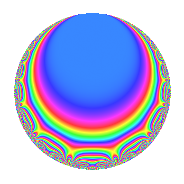# Properties

 Label 189.2.p.cLevel 189 Weight 2 Character orbit 189.p Analytic conductor 1.509 Analytic rank 0 Dimension 4 CM No Inner twists 4

# Learn more about

## Newspace parameters

 Level: $$N$$ = $$189 = 3^{3} \cdot 7$$ Weight: $$k$$ = $$2$$ Character orbit: $$[\chi]$$ = 189.p (of order $$6$$ and degree $$2$$)

## Newform invariants

 Self dual: No Analytic conductor: $$1.5091725982$$ Analytic rank: $$0$$ Dimension: $$4$$ Relative dimension: $$2$$ over $$\Q(\zeta_{6})$$ Coefficient field: $$\Q(\sqrt{-3}, \sqrt{-5})$$ Coefficient ring: $$\Z[a_1, \ldots, a_{4}]$$ Coefficient ring index: $$1$$ Sato-Tate group: $\mathrm{SU}(2)[C_{6}]$

## $q$-expansion

Coefficients of the $$q$$-expansion are expressed in terms of a basis $$1,\beta_1,\beta_2,\beta_3$$ for the coefficient ring described below. We also show the integral $$q$$-expansion of the trace form.

 $$f(q)$$ $$=$$ $$q$$ $$+ \beta_{1} q^{2}$$ $$+ 3 \beta_{2} q^{4}$$ $$+ ( -3 + 2 \beta_{2} ) q^{7}$$ $$+ \beta_{3} q^{8}$$ $$+O(q^{10})$$ $$q$$ $$+ \beta_{1} q^{2}$$ $$+ 3 \beta_{2} q^{4}$$ $$+ ( -3 + 2 \beta_{2} ) q^{7}$$ $$+ \beta_{3} q^{8}$$ $$+ ( 2 \beta_{1} - 2 \beta_{3} ) q^{11}$$ $$+ ( -1 + 2 \beta_{2} ) q^{13}$$ $$+ ( -3 \beta_{1} + 2 \beta_{3} ) q^{14}$$ $$+ ( 1 - \beta_{2} ) q^{16}$$ $$+ ( -2 \beta_{1} - 2 \beta_{3} ) q^{17}$$ $$+ ( -2 - 2 \beta_{2} ) q^{19}$$ $$+ 10 q^{22}$$ $$-2 \beta_{1} q^{23}$$ $$+ 5 \beta_{2} q^{25}$$ $$+ ( -\beta_{1} + 2 \beta_{3} ) q^{26}$$ $$+ ( -6 - 3 \beta_{2} ) q^{28}$$ $$+ 2 \beta_{3} q^{29}$$ $$+ ( -2 + \beta_{2} ) q^{31}$$ $$+ ( 3 \beta_{1} - 3 \beta_{3} ) q^{32}$$ $$+ ( 10 - 20 \beta_{2} ) q^{34}$$ $$+ ( -5 + 5 \beta_{2} ) q^{37}$$ $$+ ( -2 \beta_{1} - 2 \beta_{3} ) q^{38}$$ $$+ ( 4 \beta_{1} - 2 \beta_{3} ) q^{41}$$ $$-7 q^{43}$$ $$+ 6 \beta_{1} q^{44}$$ $$-10 \beta_{2} q^{46}$$ $$+ ( -2 \beta_{1} + 4 \beta_{3} ) q^{47}$$ $$+ ( 5 - 8 \beta_{2} ) q^{49}$$ $$+ 5 \beta_{3} q^{50}$$ $$+ ( -6 + 3 \beta_{2} ) q^{52}$$ $$+ ( 2 \beta_{1} - 2 \beta_{3} ) q^{53}$$ $$+ ( -2 \beta_{1} - \beta_{3} ) q^{56}$$ $$+ ( -10 + 10 \beta_{2} ) q^{58}$$ $$+ ( 2 \beta_{1} + 2 \beta_{3} ) q^{59}$$ $$+ ( 5 + 5 \beta_{2} ) q^{61}$$ $$+ ( -2 \beta_{1} + \beta_{3} ) q^{62}$$ $$+ 13 q^{64}$$ $$+ \beta_{2} q^{67}$$ $$+ ( 6 \beta_{1} - 12 \beta_{3} ) q^{68}$$ $$-4 \beta_{3} q^{71}$$ $$+ ( 8 - 4 \beta_{2} ) q^{73}$$ $$+ ( -5 \beta_{1} + 5 \beta_{3} ) q^{74}$$ $$+ ( 6 - 12 \beta_{2} ) q^{76}$$ $$+ ( -2 \beta_{1} + 6 \beta_{3} ) q^{77}$$ $$+ ( -11 + 11 \beta_{2} ) q^{79}$$ $$+ ( 10 + 10 \beta_{2} ) q^{82}$$ $$+ ( -4 \beta_{1} + 2 \beta_{3} ) q^{83}$$ $$-7 \beta_{1} q^{86}$$ $$+ 10 \beta_{2} q^{88}$$ $$+ ( -4 \beta_{1} + 8 \beta_{3} ) q^{89}$$ $$+ ( -1 - 4 \beta_{2} ) q^{91}$$ $$-6 \beta_{3} q^{92}$$ $$+ ( -20 + 10 \beta_{2} ) q^{94}$$ $$+ ( 1 - 2 \beta_{2} ) q^{97}$$ $$+ ( 5 \beta_{1} - 8 \beta_{3} ) q^{98}$$ $$+O(q^{100})$$ $$\operatorname{Tr}(f)(q)$$ $$=$$ $$4q$$ $$\mathstrut +\mathstrut 6q^{4}$$ $$\mathstrut -\mathstrut 8q^{7}$$ $$\mathstrut +\mathstrut O(q^{10})$$ $$4q$$ $$\mathstrut +\mathstrut 6q^{4}$$ $$\mathstrut -\mathstrut 8q^{7}$$ $$\mathstrut +\mathstrut 2q^{16}$$ $$\mathstrut -\mathstrut 12q^{19}$$ $$\mathstrut +\mathstrut 40q^{22}$$ $$\mathstrut +\mathstrut 10q^{25}$$ $$\mathstrut -\mathstrut 30q^{28}$$ $$\mathstrut -\mathstrut 6q^{31}$$ $$\mathstrut -\mathstrut 10q^{37}$$ $$\mathstrut -\mathstrut 28q^{43}$$ $$\mathstrut -\mathstrut 20q^{46}$$ $$\mathstrut +\mathstrut 4q^{49}$$ $$\mathstrut -\mathstrut 18q^{52}$$ $$\mathstrut -\mathstrut 20q^{58}$$ $$\mathstrut +\mathstrut 30q^{61}$$ $$\mathstrut +\mathstrut 52q^{64}$$ $$\mathstrut +\mathstrut 2q^{67}$$ $$\mathstrut +\mathstrut 24q^{73}$$ $$\mathstrut -\mathstrut 22q^{79}$$ $$\mathstrut +\mathstrut 60q^{82}$$ $$\mathstrut +\mathstrut 20q^{88}$$ $$\mathstrut -\mathstrut 12q^{91}$$ $$\mathstrut -\mathstrut 60q^{94}$$ $$\mathstrut +\mathstrut O(q^{100})$$

Basis of coefficient ring in terms of a root $$\nu$$ of $$x^{4}\mathstrut -\mathstrut$$ $$5$$ $$x^{2}\mathstrut +\mathstrut$$ $$25$$:

 $$\beta_{0}$$ $$=$$ $$1$$ $$\beta_{1}$$ $$=$$ $$\nu$$ $$\beta_{2}$$ $$=$$ $$\nu^{2}$$$$/5$$ $$\beta_{3}$$ $$=$$ $$\nu^{3}$$$$/5$$
 $$1$$ $$=$$ $$\beta_0$$ $$\nu$$ $$=$$ $$\beta_{1}$$ $$\nu^{2}$$ $$=$$ $$5$$ $$\beta_{2}$$ $$\nu^{3}$$ $$=$$ $$5$$ $$\beta_{3}$$

## Character Values

We give the values of $$\chi$$ on generators for $$\left(\mathbb{Z}/189\mathbb{Z}\right)^\times$$.

 $$n$$ $$29$$ $$136$$ $$\chi(n)$$ $$-1$$ $$1 - \beta_{2}$$

## Embeddings

For each embedding $$\iota_m$$ of the coefficient field, the values $$\iota_m(a_n)$$ are shown below.

For more information on an embedded modular form you can click on its label.

Label $$\iota_m(\nu)$$ $$a_{2}$$ $$a_{3}$$ $$a_{4}$$ $$a_{5}$$ $$a_{6}$$ $$a_{7}$$ $$a_{8}$$ $$a_{9}$$ $$a_{10}$$
26.1
 −1.93649 − 1.11803i 1.93649 + 1.11803i −1.93649 + 1.11803i 1.93649 − 1.11803i
−1.93649 1.11803i 0 1.50000 + 2.59808i 0 0 −2.00000 + 1.73205i 2.23607i 0 0
26.2 1.93649 + 1.11803i 0 1.50000 + 2.59808i 0 0 −2.00000 + 1.73205i 2.23607i 0 0
80.1 −1.93649 + 1.11803i 0 1.50000 2.59808i 0 0 −2.00000 1.73205i 2.23607i 0 0
80.2 1.93649 1.11803i 0 1.50000 2.59808i 0 0 −2.00000 1.73205i 2.23607i 0 0
 $$n$$: e.g. 2-40 or 990-1000 Significant digits: Format: Complex embeddings Normalized embeddings Satake parameters Satake angles

## Inner twists

Char. orbit Parity Mult. Self Twist Proved
1.a Even 1 trivial yes
3.b Odd 1 yes
7.d Odd 1 yes
21.g Even 1 yes

## Hecke kernels

This newform can be constructed as the kernel of the linear operator $$T_{2}^{4}$$ $$\mathstrut -\mathstrut 5 T_{2}^{2}$$ $$\mathstrut +\mathstrut 25$$ acting on $$S_{2}^{\mathrm{new}}(189, [\chi])$$.